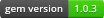# Liblinear-RubyLiblinear-Ruby is Ruby interface of LIBLINEAR using SWIG.
Now, this interface is supporting LIBLINEAR 2.1.

## Installation

``````gem 'liblinear-ruby'
``````

And then execute:

``````\$ bundle
``````

Or install it yourself as:

``````\$ gem install liblinear-ruby
``````

## Quick Start

This sample code execute classification with L2-regularized logistic regression.

``````require 'liblinear'

# train
model = Liblinear.train(
{ solver_type: Liblinear::L2R_LR },   # parameter
[-1, -1, 1, 1],                       # labels (classes) of training data
[[-2, -2], [-1, -1], [1, 1], [2, 2]], # training data
)
# predict
puts Liblinear.predict(model, [0.5, 0.5]) # predicted class will be 1
``````

## Parameter

There are some parameters you can specify:

• `solver_type`
• `cost`
• `sensitive_loss`
• `epsilon`
• `weight_labels` and `weights`

### solver_type

This parameter specifies a type of solver (default: `Liblinear::L2R_L2LOSS_SVC_DUAL`).
This corresponds to `-s` option on command line.
Solver types you can set are shown below:

``````# for multi-class classification
Liblinear::L2R_LR              # L2-regularized logistic regression (primal)
Liblinear::L2R_L2LOSS_SVC_DUAL # L2-regularized L2-loss support vector classification (dual)
Liblinear::L2R_L2LOSS_SVC      # L2-regularized L2-loss support vector classification (primal)
Liblinear::L2R_L1LOSS_SVC_DUAL # L2-regularized L1-loss support vector classification (dual)
Liblinear::MCSVM_CS            # support vector classification by Crammer and Singer
Liblinear::L1R_L2LOSS_SVC      # L1-regularized L2-loss support vector classification
Liblinear::L1R_LR              # L1-regularized logistic regression
Liblinear::L2R_LR_DUAL         # L2-regularized logistic regression (dual)

# for regression
Liblinear::L2R_L2LOSS_SVR      # L2-regularized L2-loss support vector regression (primal)
Liblinear::L2R_L2LOSS_SVR_DUAL # L2-regularized L2-loss support vector regression (dual)
Liblinear::L2R_L1LOSS_SVR_DUAL # L2-regularized L1-loss support vector regression (dual)
``````

### cost

This parameter specifies the cost of constraints violation (default `1.0`).
This corresponds to `-c` option on command line.

### sensitive_loss

This parameter specifies an epsilon in loss function of epsilon-SVR (default `0.1`).
This corresponds to `-p` option on command line.

### epsilon

This parameter specifies a tolerance of termination criterion.
This corresponds to `-e` option on command line.
The default value depends on a type of solver. See LIBLINEAR's README or `Liblinear::Parameter.default_epsion` for more details.

### weight_labels and weights

These parameters are used to change the penalty for some classes (default `[]`).
Each `weights[i]` corresponds to `weight_labels[i]`, meaning that the penalty of class `weight_labels[i]` is scaled by a factor of `weights[i]`.

## Train

First, prepare training data.

``````# Define class of each training data:
labels = [1, -1, ...]

# Training data is Array of Array:
examples = [
[1, 0, 0, 1, 0],
[0, 0, 0, 1, 1],
...
]

# You can also use Array of Hash instead:
examples = [
{ 1 => 1, 4 => 1 },
{ 4 => 1, 5 => 1 },
...
]
``````

Next, set the bias (this corresponds to `-B` option on command line):

``````bias = 0.5 # default -1
``````

Then, specify parameters and execute `Liblinear.train` to get the instance of `Liblinear::Model`.

``````model = Liblinear.train(parameter, labels, examples, bias)
``````

In this phase, you can save model as:

``````model.save(file_name)
``````

``````model = Liblinear::Model.load(file_name)
``````

## Predict

Prepare the data you want to predict its class and call `Liblinear.predict`.

``````examples = [0, 0, 0, 1, 1]
Liblinear.predict(model, example)
``````

## Cross Validation

To get classes predicted by k-fold cross validation, use `Liblinear.cross_validation`.
For example, `results` is a class predicted by `examples` excepts part including `examples`.

``````results = Liblinear.cross_validation(fold, parameter, labels, examples)
``````